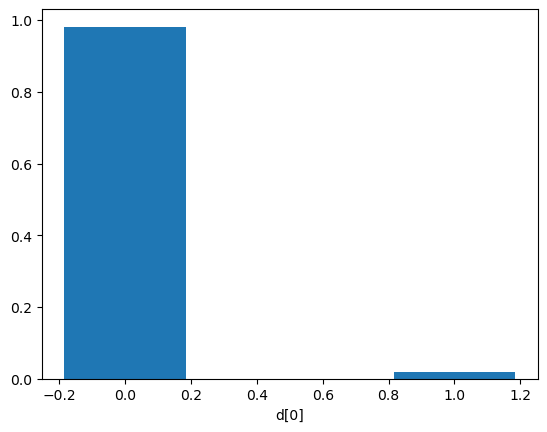# I - Model comparison#

Authors: Noemi Anau Montel, James Alvey, Christoph Weniger

Last update: 15 September 2023

Proposal: How to perform model comparison in swyft estimating the Bayes factor.

Key take-away messages: Learn how to use the switch method to introduce conditional dependency in the computational graph.

## Code#

:

import numpy as np
from scipy import stats
import pylab as plt
import torch
import swyft
DEVICE = 'gpu' if torch.cuda.is_available() else 'cpu'

:

torch.manual_seed(0)
np.random.seed(0)


Although we cannot direclty estimate the evidence of data, $$p(\mathbf x)$$, given a model, we can use neural ratio estimation to estimate the ratio of two marginal likelihoods, also known as the Bayes factor.

If we have to models $$M0$$ and $$M1$$, we can estimate the Bayes factor

$K = \frac{p(\mathbf x|M0)}{p(\mathbf x|M1)}$

by taking the ratio of the ratios

$r(\mathbf x; M0) = \frac{p(\mathbf x|M0)}{p(\mathbf x)}$

and

$r(\mathbf x; M1) = \frac{p(\mathbf x|M1)}{p(\mathbf x)}\;.$

The evidence $$p(\mathbf x)$$ would cancel out in this case.

As an example, let us consider two simulators. We then define a composite simulator which has as its only parameter a discrete value that decides which of the base simulators to use. This exploits the fact that simulators can be nested in Swyft.

:

class Sim_M0(swyft.Simulator):
def __init__(self):
super().__init__()
self.transform_samples = swyft.to_numpy32

def build(self, graph):
x = graph.node('x', lambda: np.random.randn(10))

class Sim_M1(swyft.Simulator):
def __init__(self):
super().__init__()
self.transform_samples = swyft.to_numpy32

def build(self, graph):
x = graph.node('x', lambda: np.random.randn(10)*2.1)

class Simulator(swyft.Simulator):
def __init__(self):
super().__init__()
self.transform_samples = swyft.to_numpy32
self.sim0 = Sim_M0()
self.sim1 = Sim_M1()

def build(self, graph):
d = graph.node('d', lambda: np.random.choice(2, size=1).astype(float))
with graph.prefix("M0/"):
self.sim0.build(graph)
with graph.prefix("M1/"):
self.sim1.build(graph)
x = graph.switch('x', [graph["M0/x"], graph["M1/x"]], d)

sim = Simulator()


Note: - We use here the switch method, which introduces some conditional dependencies in the computational graph. Depending on the value of d, x is assigned different values. The switch statements make sure that parameter dependencies are properly propagated and only relevant computational nodes are executed. - We combine the graphs from multiple models by explicitly calling the build method of sub-models. - We use the prefix method to prepend some additional string to the parameter names of the included sub-models. This acts as neat namespace.

:

samples = sim.sample(10000)


Inference network and training work similar to before. (Note that for more than 2 classes, it would be a good idea to use one-hot encoding before passing parameters to the LogRatioEstimator - we do not worry about this here).

:

class Network(swyft.SwyftModule):
def __init__(self):
super().__init__()
self.logratios = swyft.LogRatioEstimator_1dim(num_features = 10, num_params = 1, varnames = 'd')

def forward(self, A, B):
logratios = self.logratios(A['x'], B['d'])
return logratios

trainer = swyft.SwyftTrainer(accelerator = DEVICE, precision = 64)
dm = swyft.SwyftDataModule(samples, batch_size = 64)
network = Network()
trainer.fit(network, dm)

GPU available: True (mps), used: False
TPU available: False, using: 0 TPU cores
IPU available: False, using: 0 IPUs
HPU available: False, using: 0 HPUs
/Users/cweniger/opt/anaconda3/envs/native2/lib/python3.9/site-packages/pytorch_lightning/trainer/setup.py:200: UserWarning: MPS available but not used. Set accelerator and devices using Trainer(accelerator='mps', devices=1).
rank_zero_warn(
/Users/cweniger/opt/anaconda3/envs/native2/lib/python3.9/site-packages/pytorch_lightning/loops/utilities.py:94: PossibleUserWarning: max_epochs was not set. Setting it to 1000 epochs. To train without an epoch limit, set max_epochs=-1.
rank_zero_warn(
The following callbacks returned in LightningModule.configure_callbacks will override existing callbacks passed to Trainer: ModelCheckpoint

| Name      | Type                   | Params
-----------------------------------------------------
0 | logratios | LogRatioEstimator_1dim | 18.0 K
-----------------------------------------------------
18.0 K    Trainable params
0         Non-trainable params
18.0 K    Total params
0.144     Total estimated model params size (MB)

/Users/cweniger/opt/anaconda3/envs/native2/lib/python3.9/site-packages/pytorch_lightning/trainer/connectors/data_connector.py:224: PossibleUserWarning: The dataloader, val_dataloader 0, does not have many workers which may be a bottleneck. Consider increasing the value of the num_workers argument (try 8 which is the number of cpus on this machine) in the DataLoader init to improve performance.
rank_zero_warn(
/Users/cweniger/opt/anaconda3/envs/native2/lib/python3.9/site-packages/pytorch_lightning/trainer/connectors/data_connector.py:224: PossibleUserWarning: The dataloader, train_dataloader, does not have many workers which may be a bottleneck. Consider increasing the value of the num_workers argument (try 8 which is the number of cpus on this machine) in the DataLoader init to improve performance.
rank_zero_warn(

Reloading best model: /Users/cweniger/Documents/swyft/notebooks/lightning_logs/version_25/checkpoints/epoch=5-step=750.ckpt


Finally, we can perform classification. As usual, we sample from the prior and infer. Then we can use the get_class_probs function to turn predictions into class probabilities.

:

obs = sim.sample()
prior_samples = sim.sample(2_000, targets = ['d'])
predictions = trainer.infer(network, obs, prior_samples)
probs = swyft.get_class_probs(predictions, 'd')

plt.bar([0, 1], probs, width = 0.37, log = False)
plt.xlabel("d");
K = probs/probs
print("True class =", obs['d'])
print("Bayes factor K = p(d=1|x)/p(d=0|x) =", K)

The following callbacks returned in LightningModule.configure_callbacks will override existing callbacks passed to Trainer: EarlyStopping, ModelCheckpoint

True class = 0.0
Bayes factor K = p(d=1|x)/p(d=0|x) = 0.018984887982884932

/Users/cweniger/opt/anaconda3/envs/native2/lib/python3.9/site-packages/pytorch_lightning/loops/epoch/prediction_epoch_loop.py:173: UserWarning: Lightning couldn't infer the indices fetched for your dataloader.[ ]:

# Code goes here